# RD Sharma Solutions for Class 12 Maths Chapter 17 Increasing and Decreasing Functions

## RD Sharma Solutions for Class 12 Maths Chapter 17 – Free PDF Download

Here RD Sharma Solutions for Class 12 Chapter 17 – Increasing and Decreasing Functions is provided for the benefit of students. RD Sharma books provide multiple-choice questions and very short answer types of questions, by which students can enhance their analytical thinking and time management skills. They have proven to be essential for learning the syllabus and building the confidence that is required to face their exams. Students are suggested to go through RD Sharma Solutions since these solutions are most repeated in the exams as well as in competitive exams.

Chapter 17 of RD Sharma Solutions for Class 12 Maths Increasing and Decreasing Functions explains monotonicity of functions. The exercise-wise solution of these topics is available here in PDF format, which can be easily downloaded by the students. The RD Sharma Solutions for Class 12 are prepared by subject experts at BYJU’S in order to guide the students in their academics.

Some of the essential topics of this chapter’s RD Sharma Solutions are listed below.

• The solution of rational algebraic inequations
• Strictly increasing functions
• Strictly decreasing functions
• Monotonic functions
• Monotonically increasing function
• Monotonically decreasing functions
• Necessary and sufficient conditions for monotonicity
• Finding the intervals in which a function is increasing or decreasing
• Proving the monotonicity of a function on a given interval
• Finding the interval in which a function is increasing or decreasing

## RD Sharma Solutions Class 12 Maths Chapter 17 Increasing and Decreasing Functions: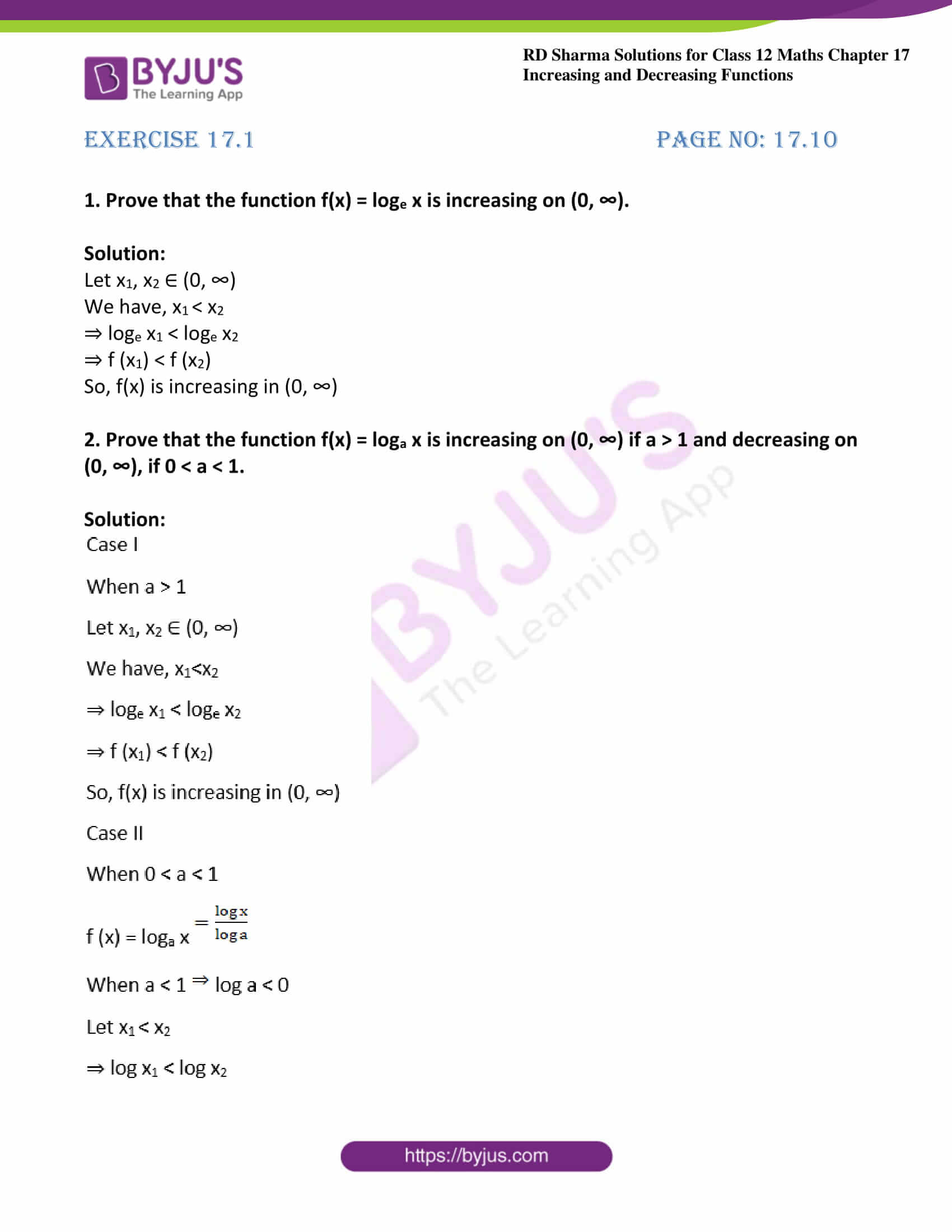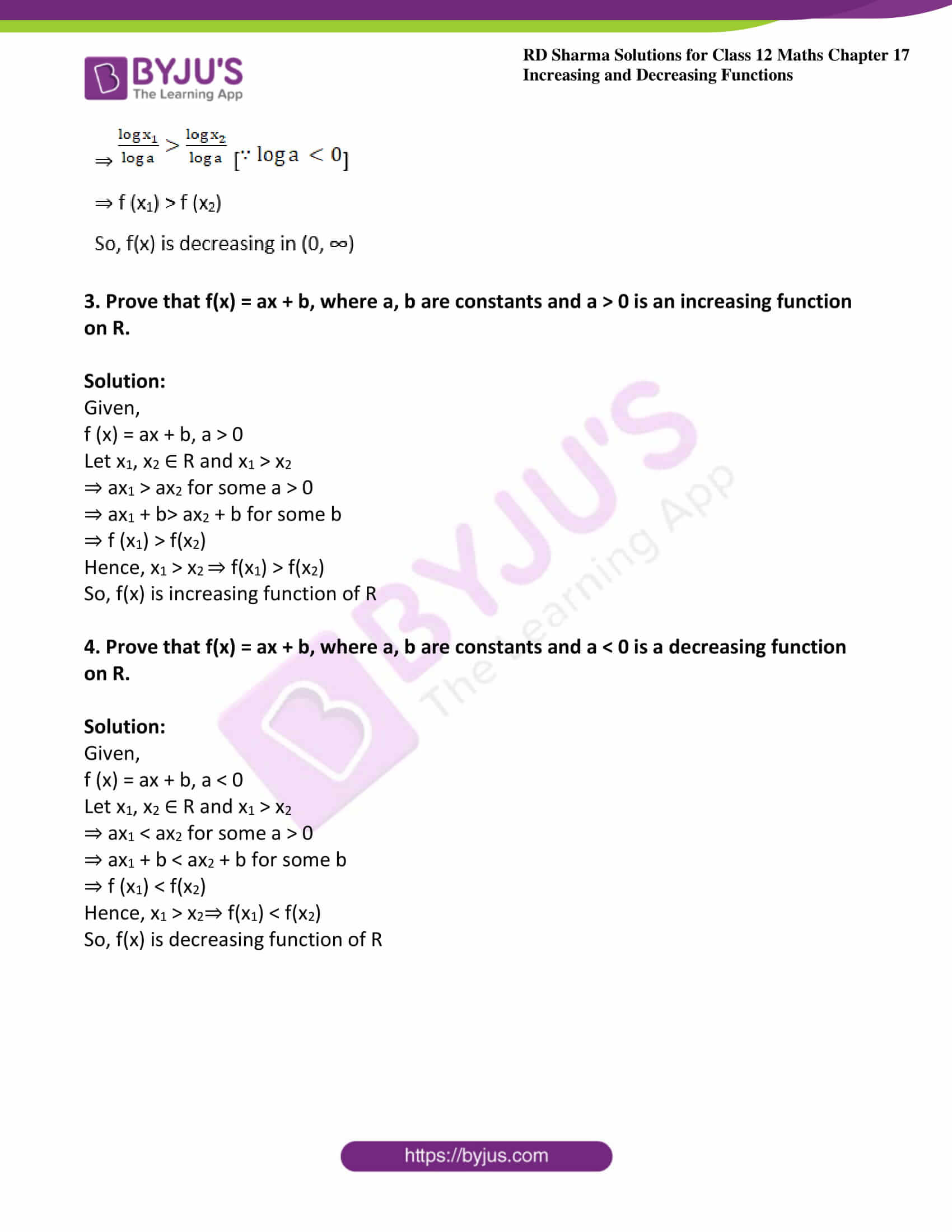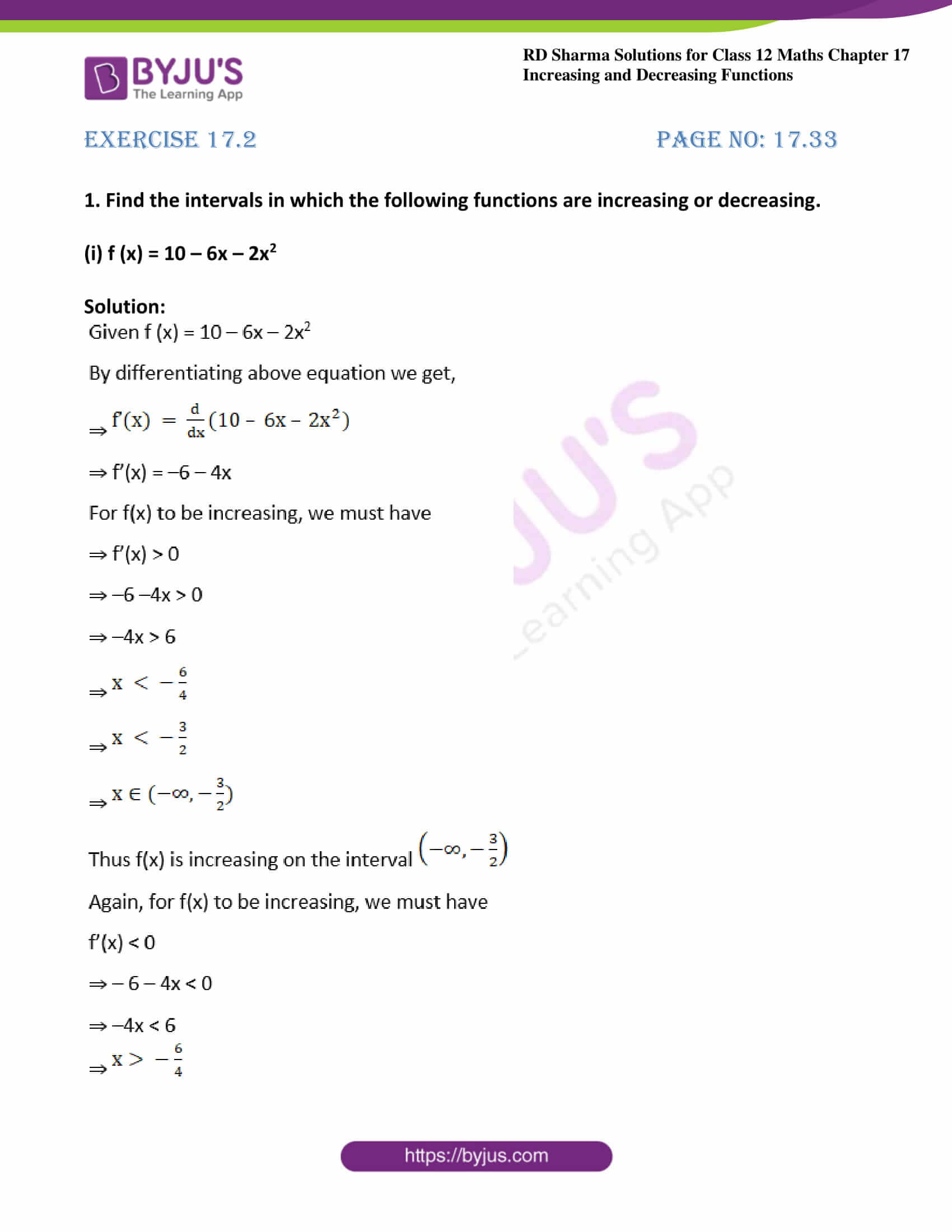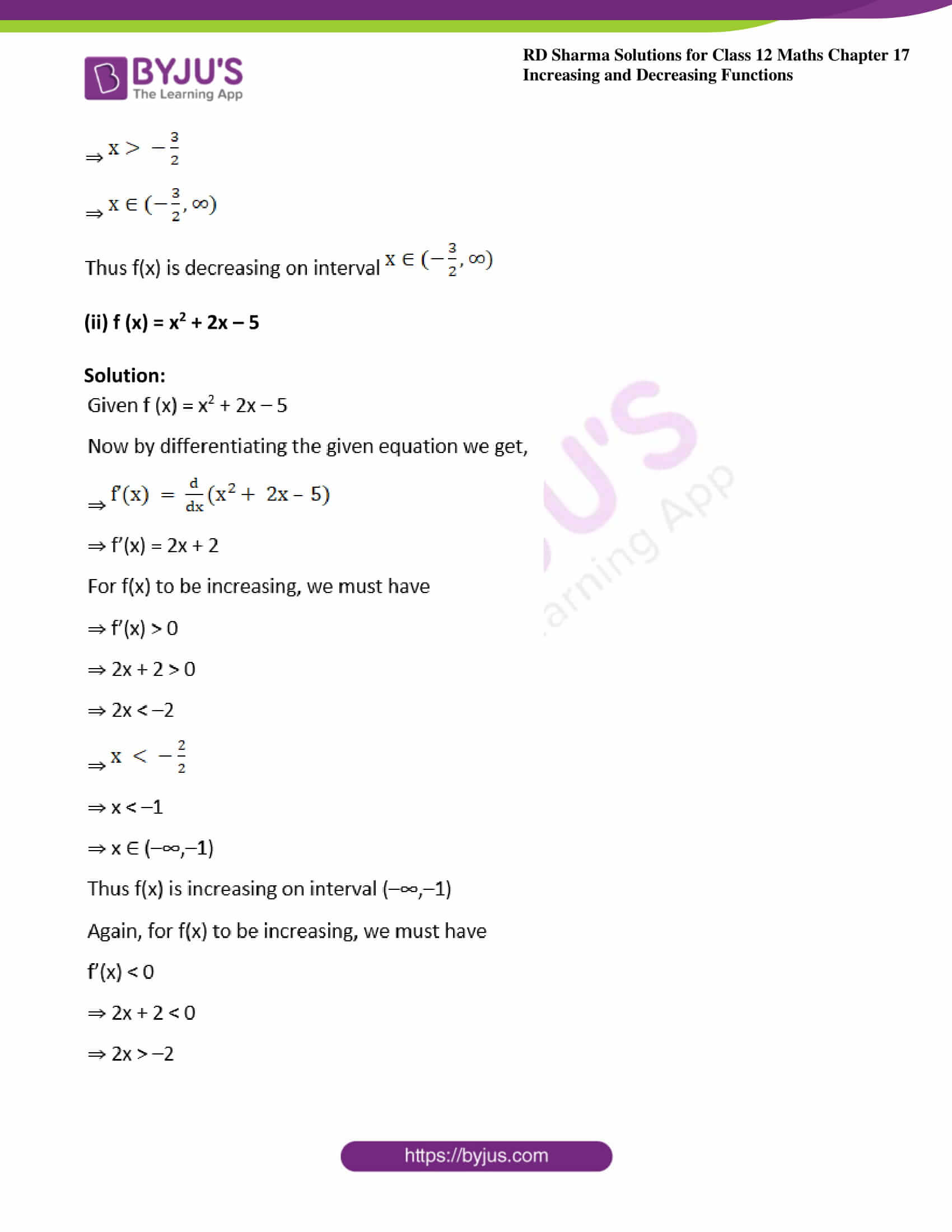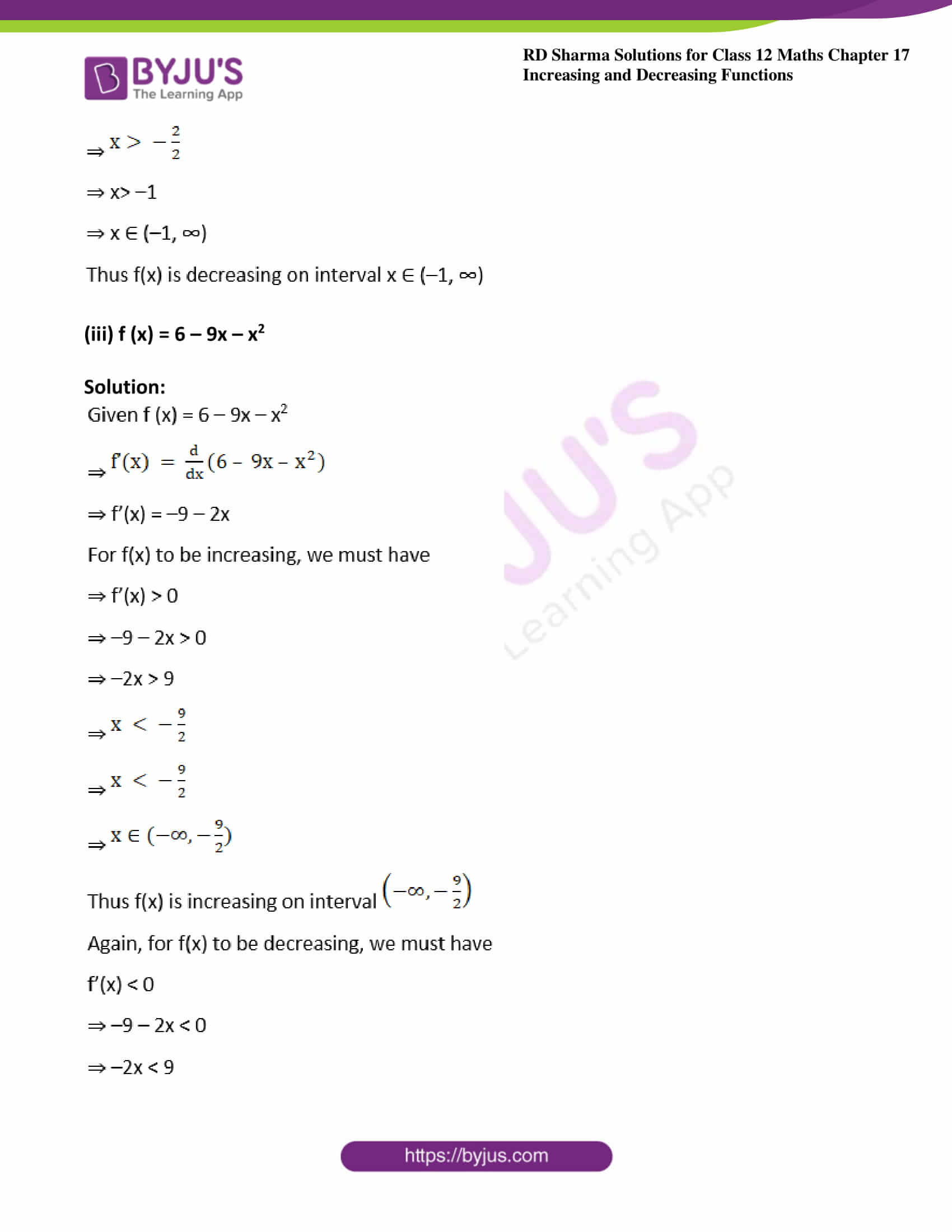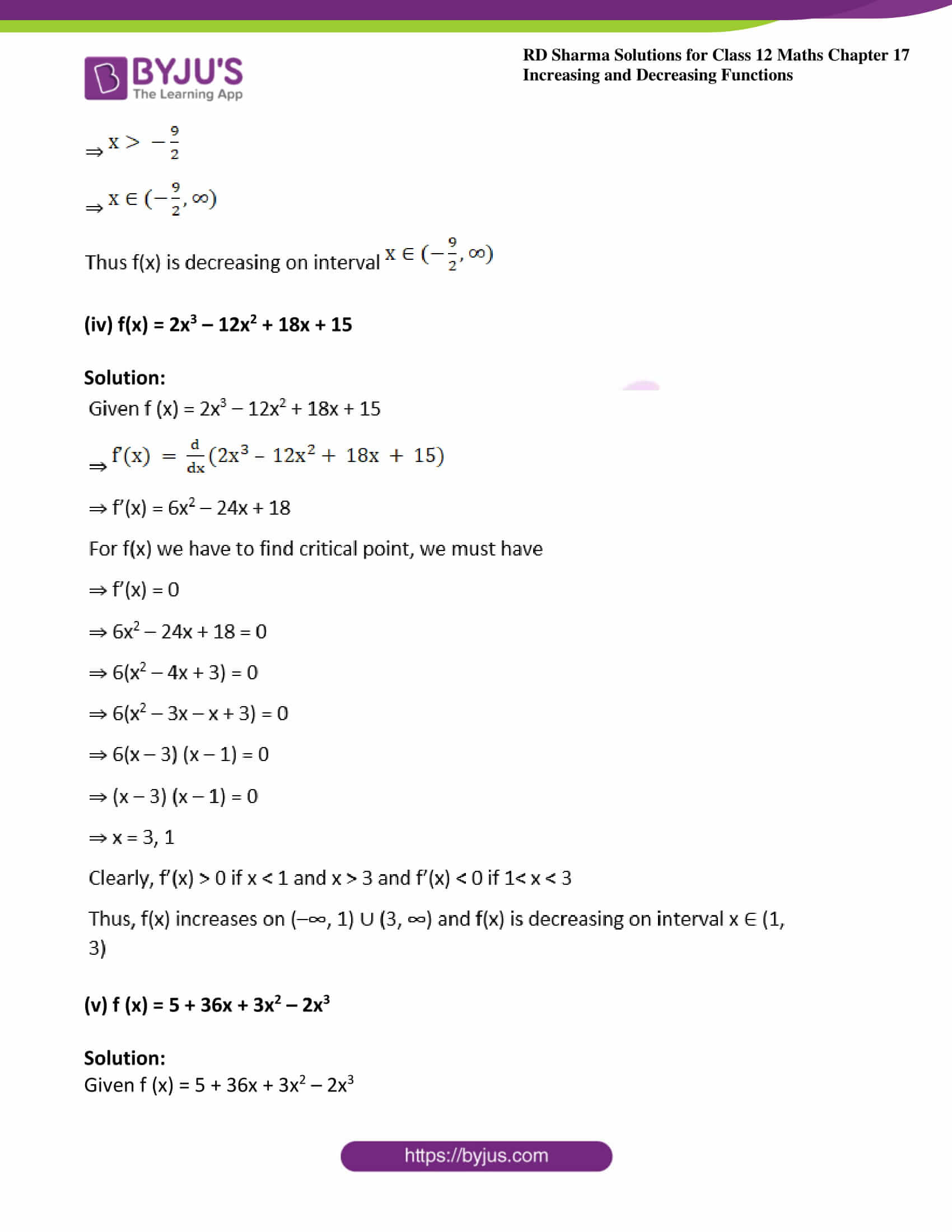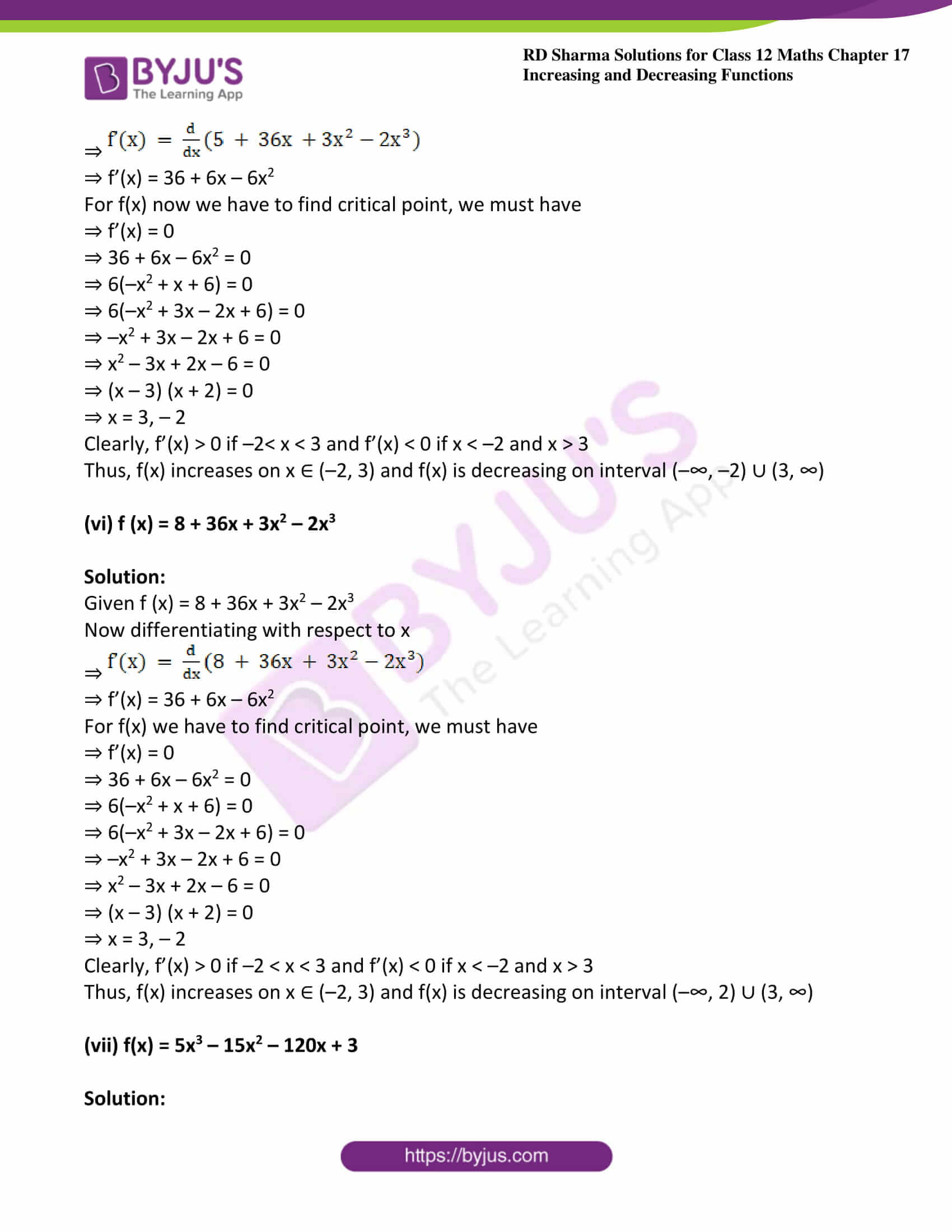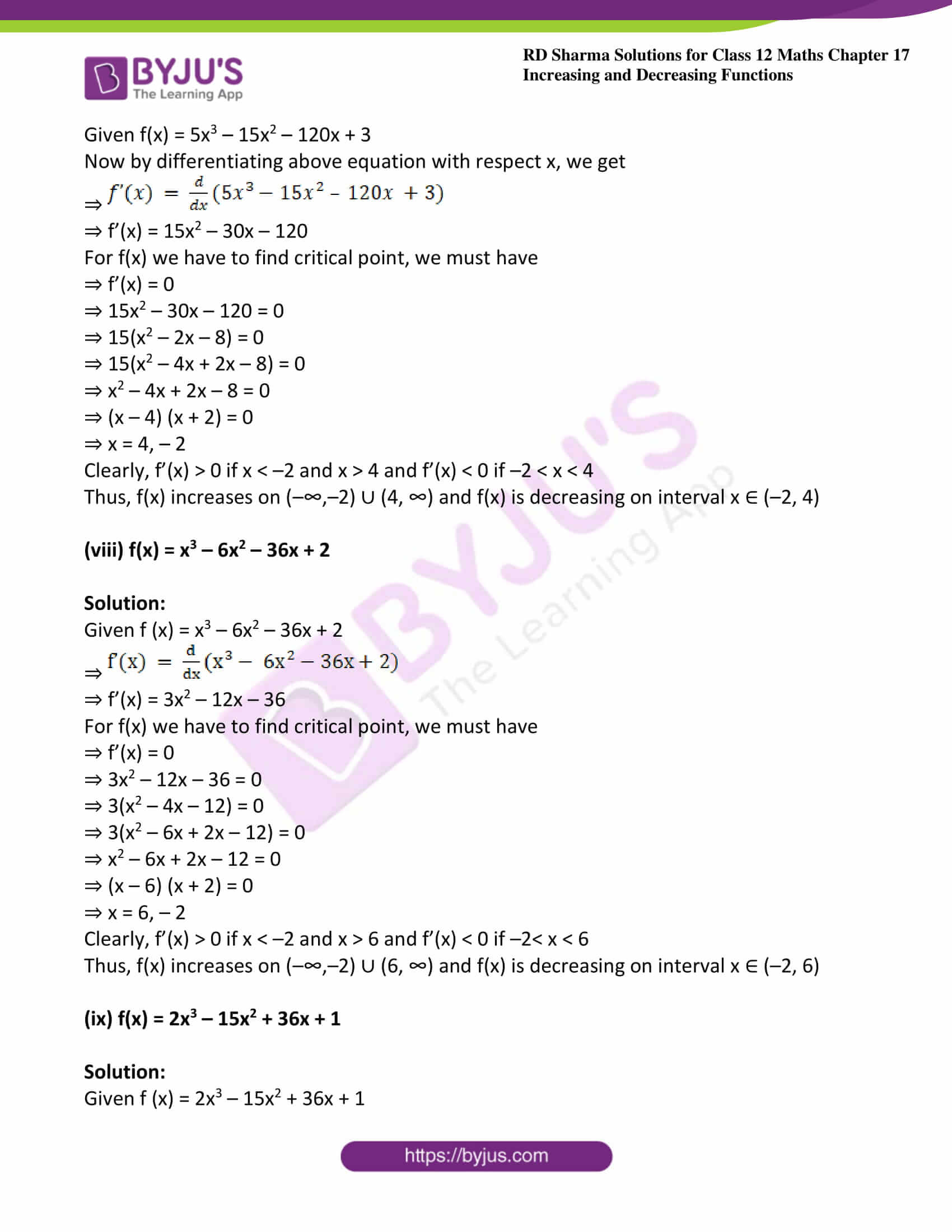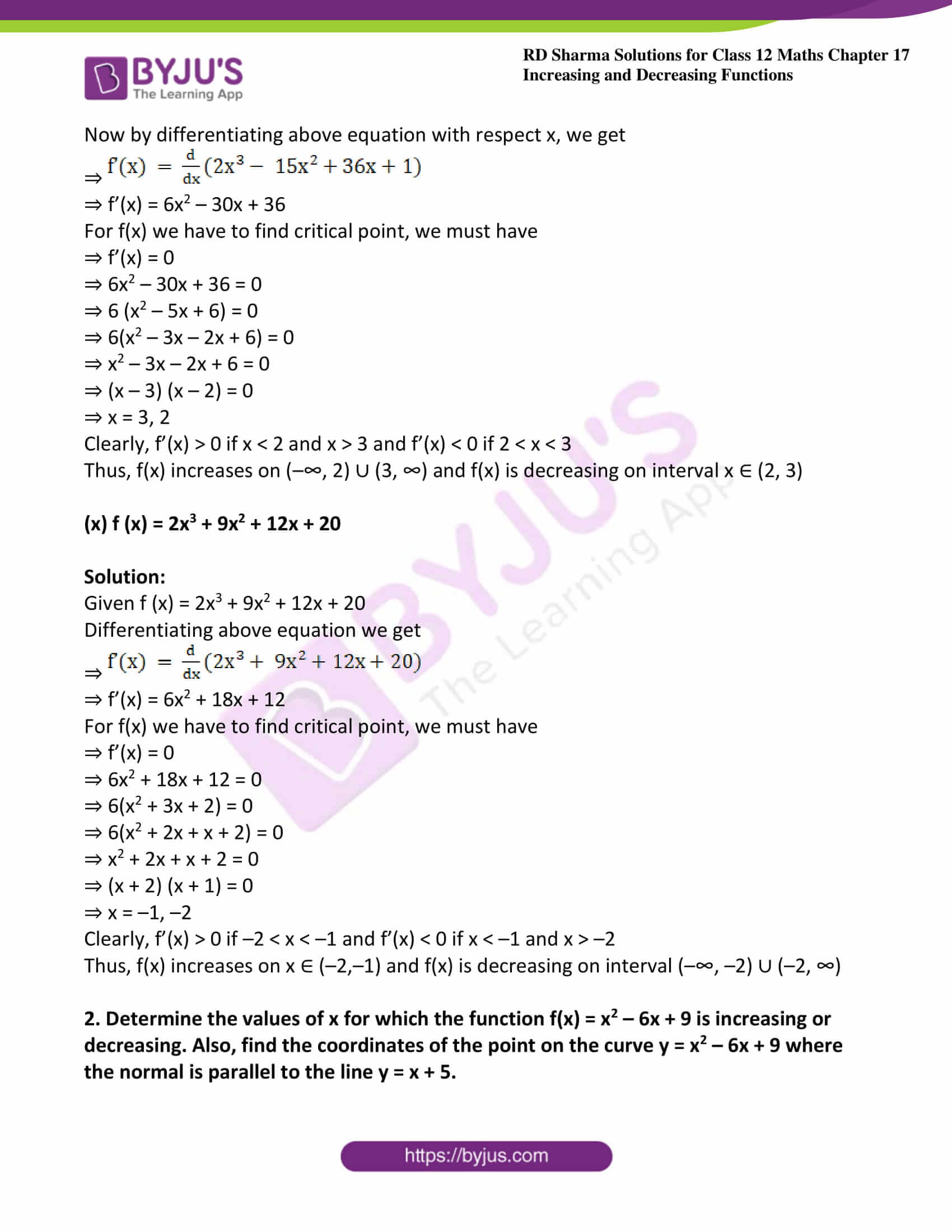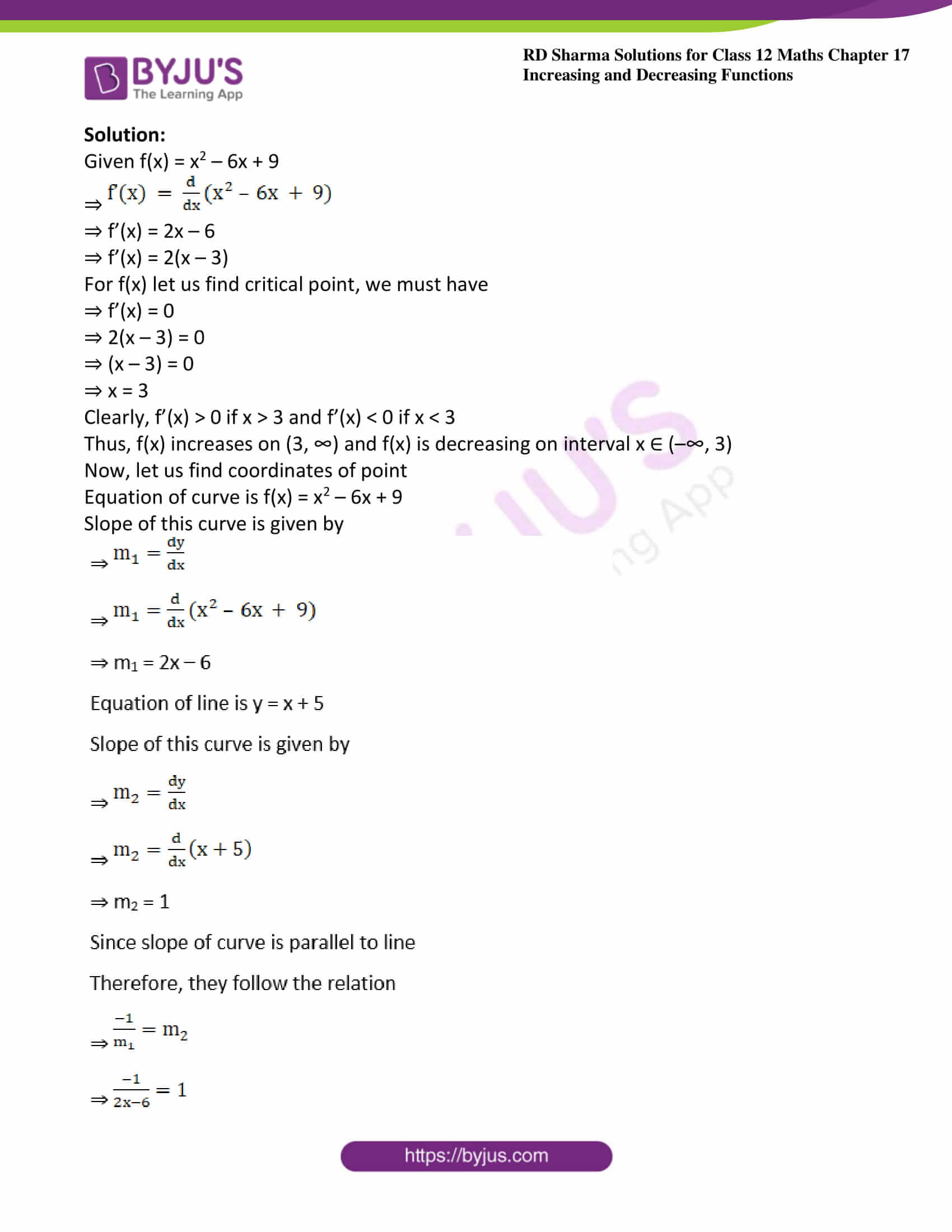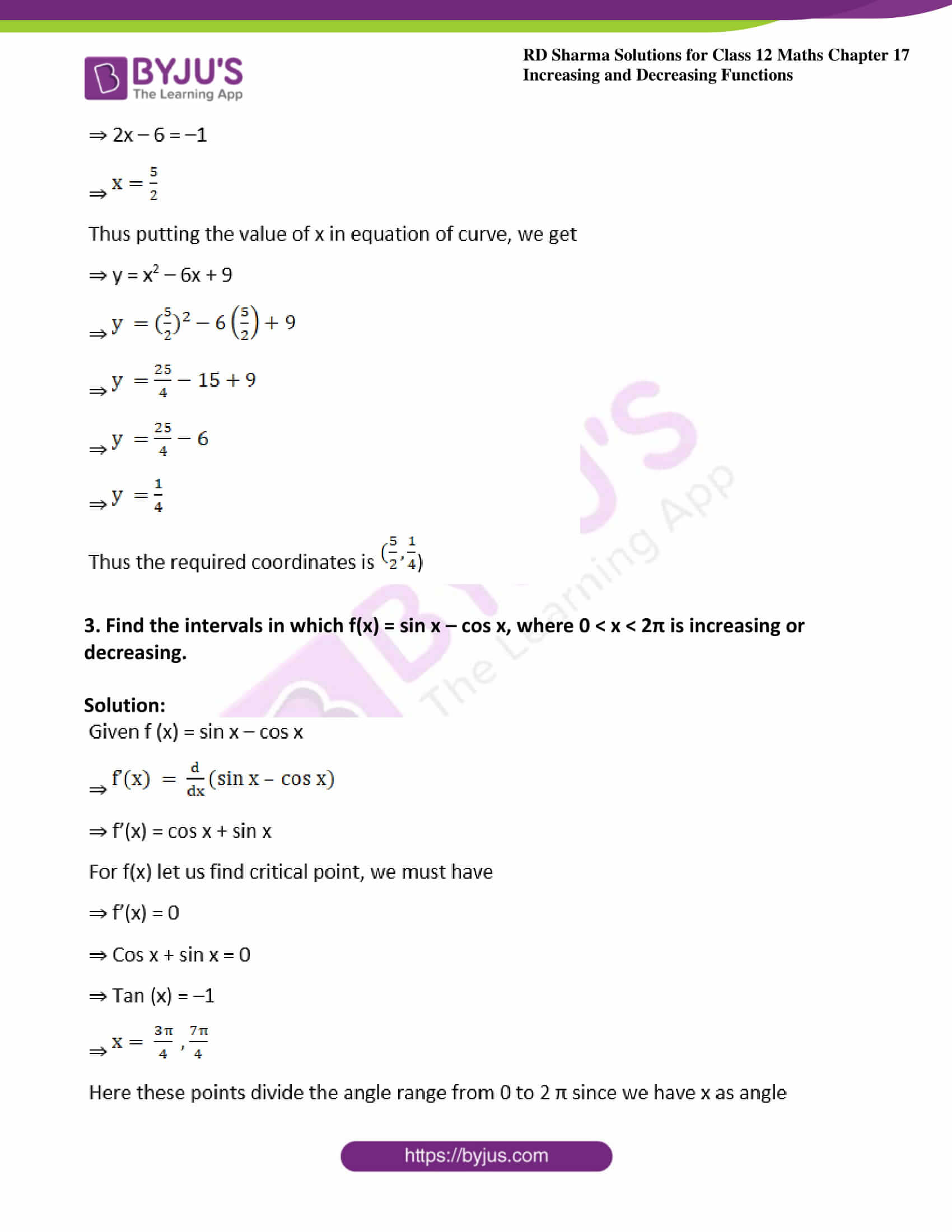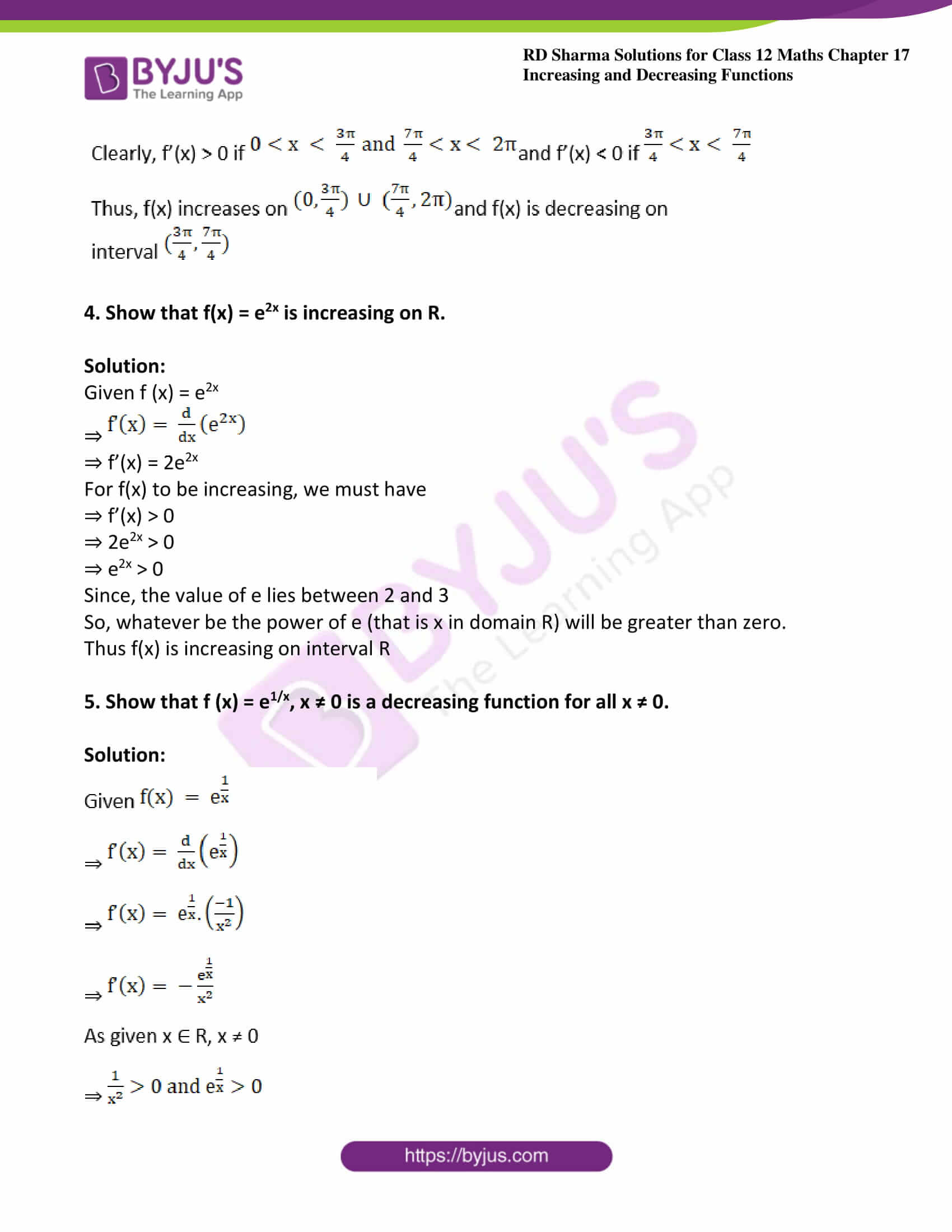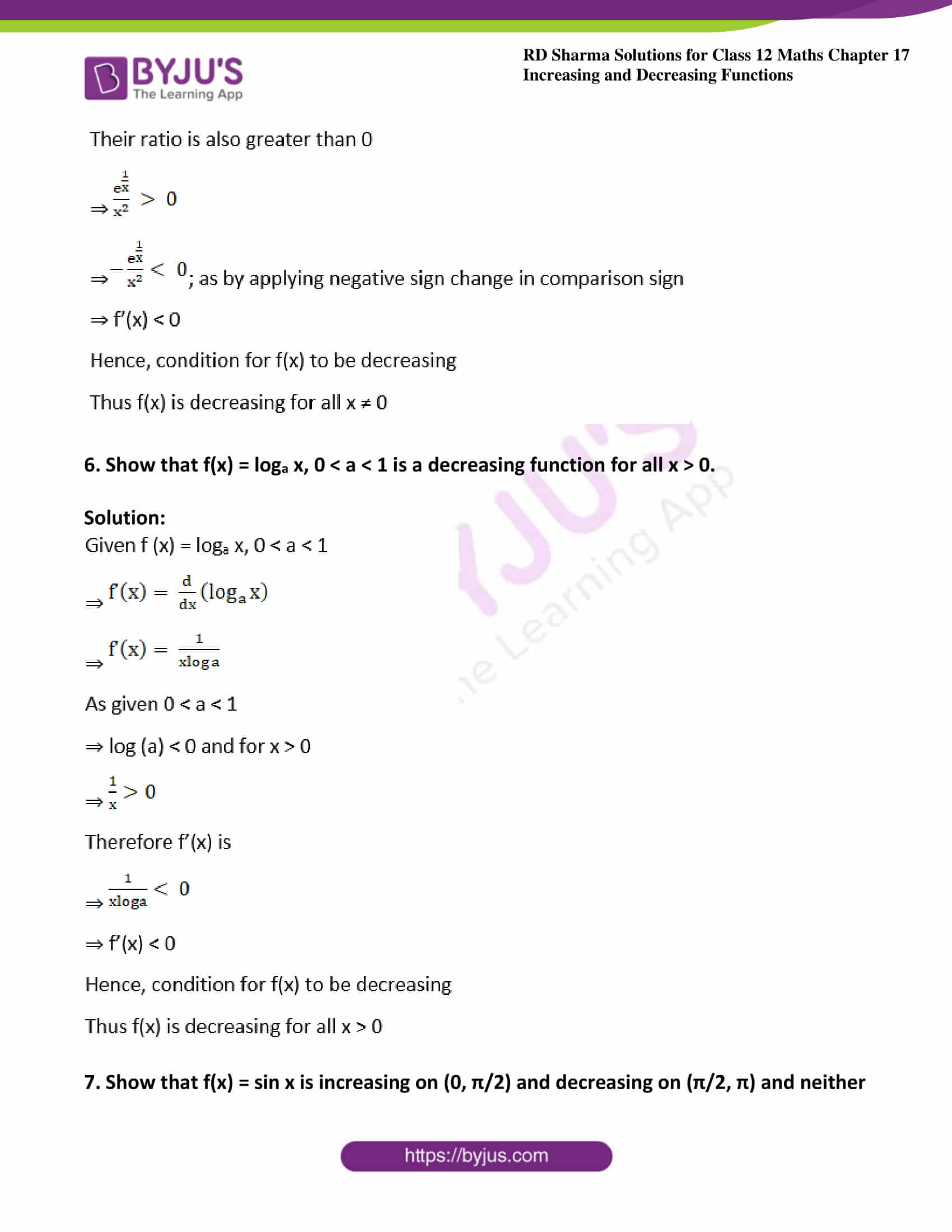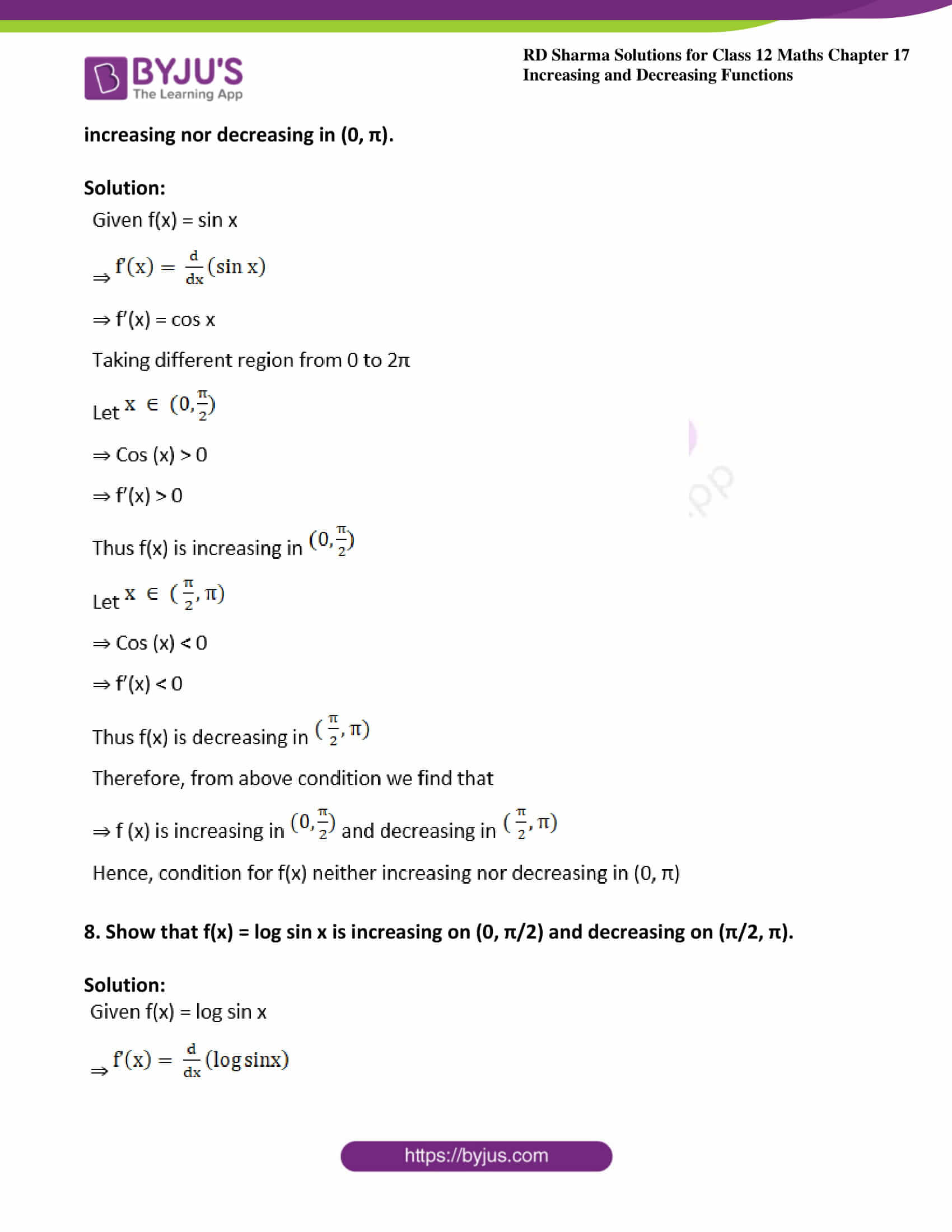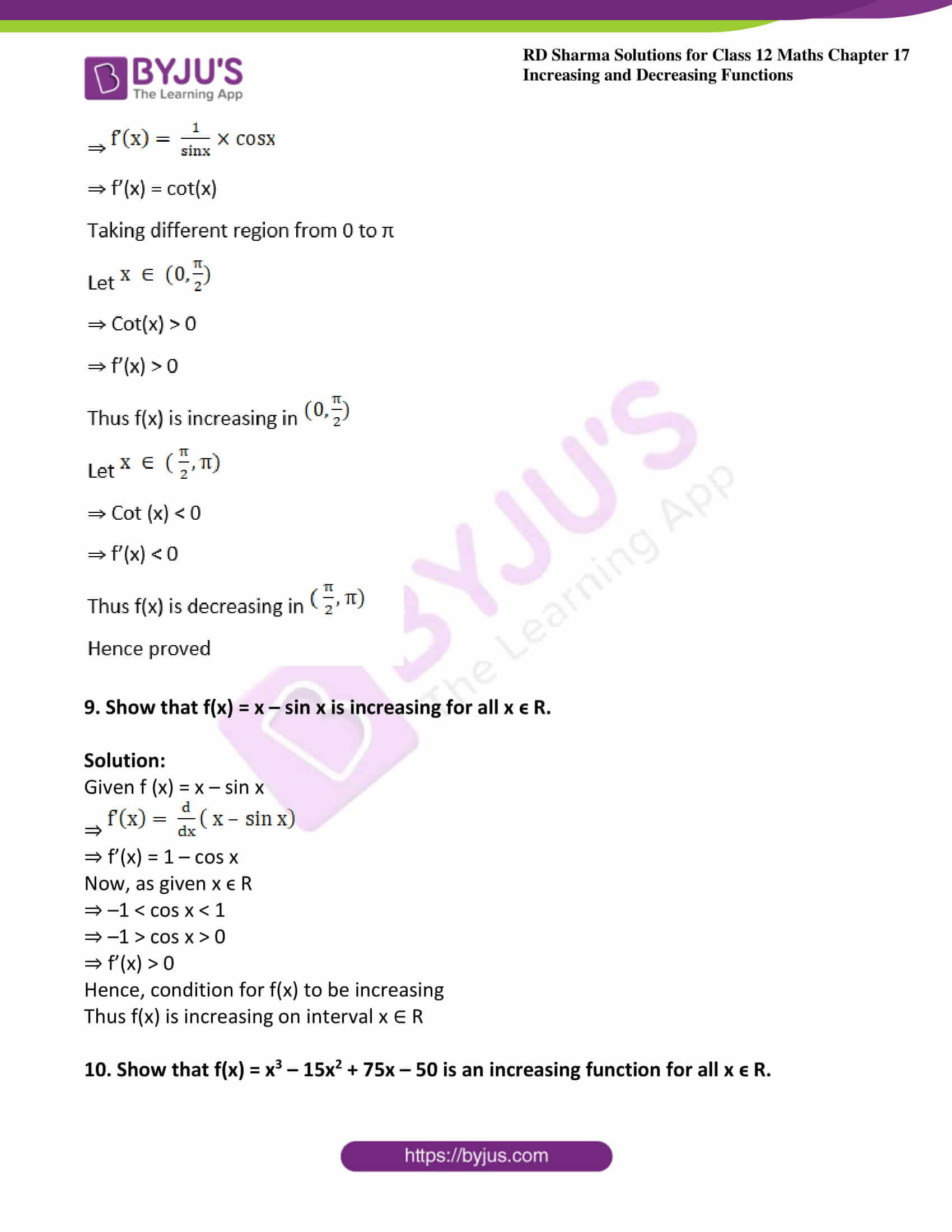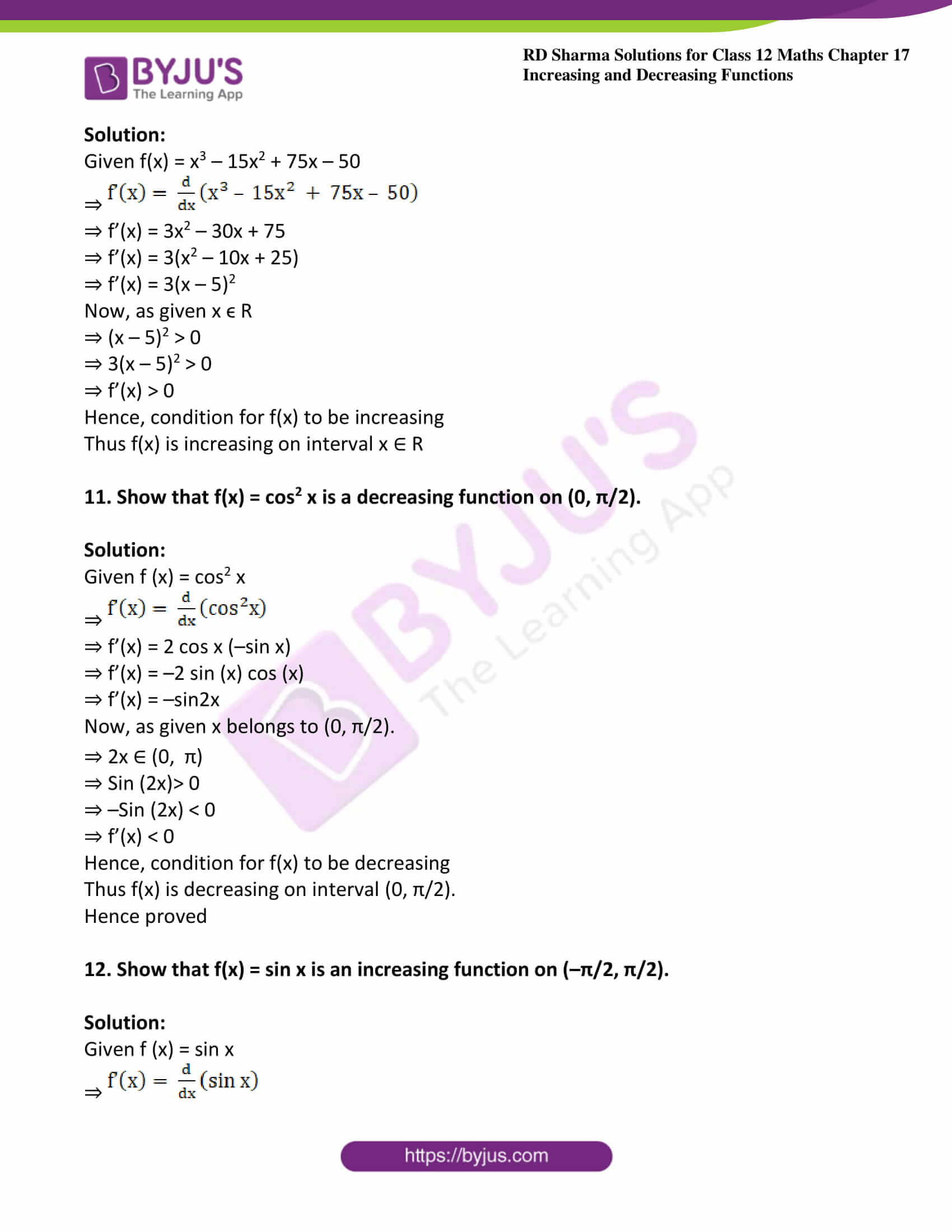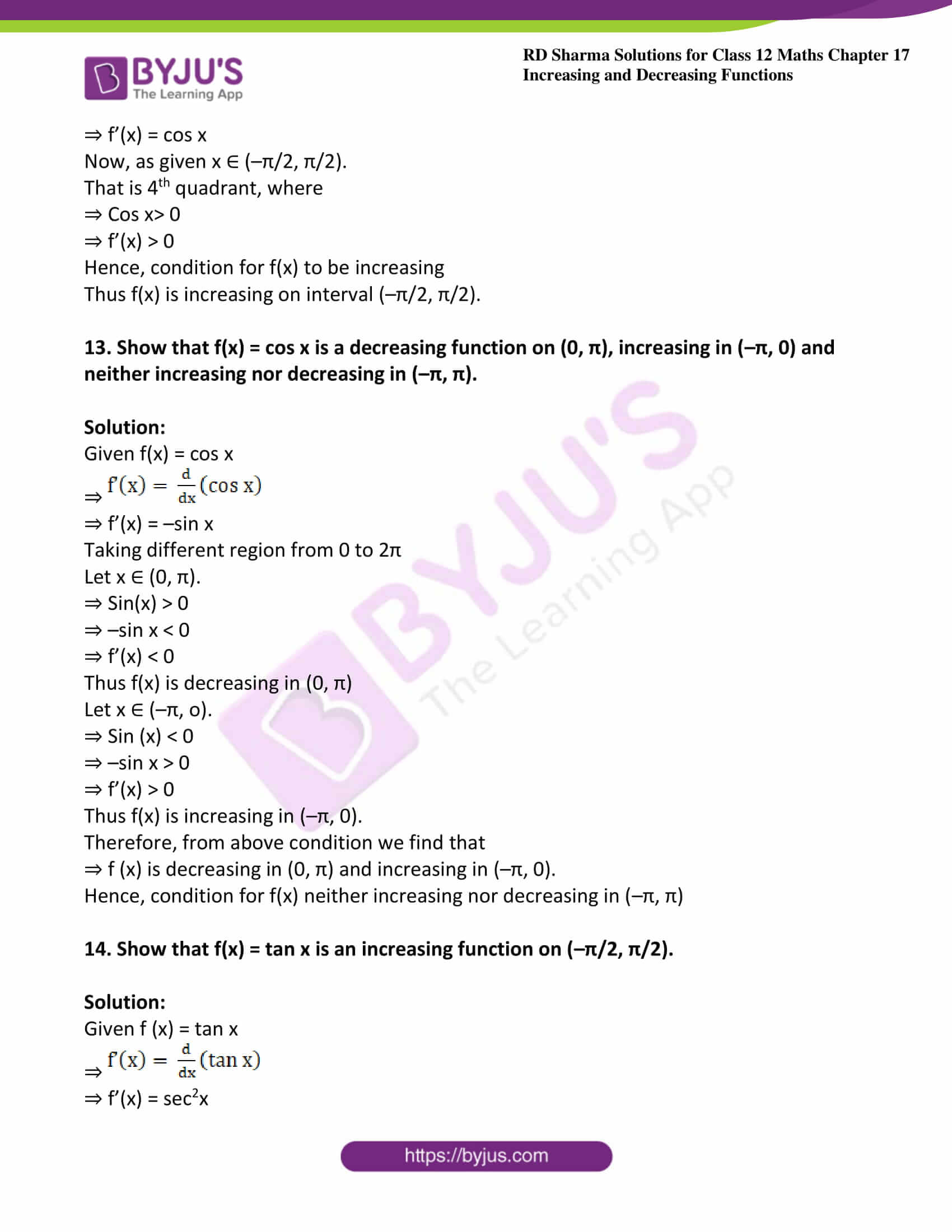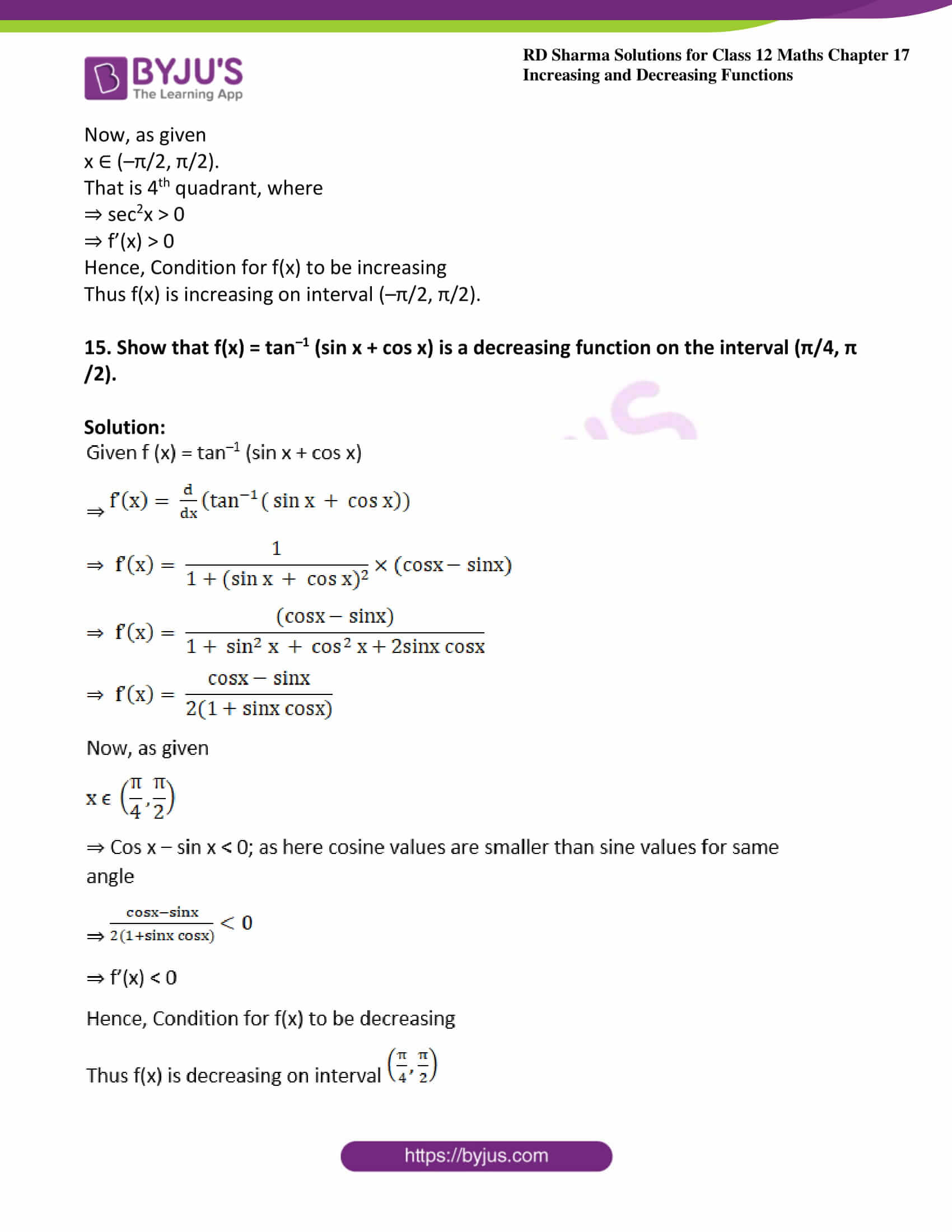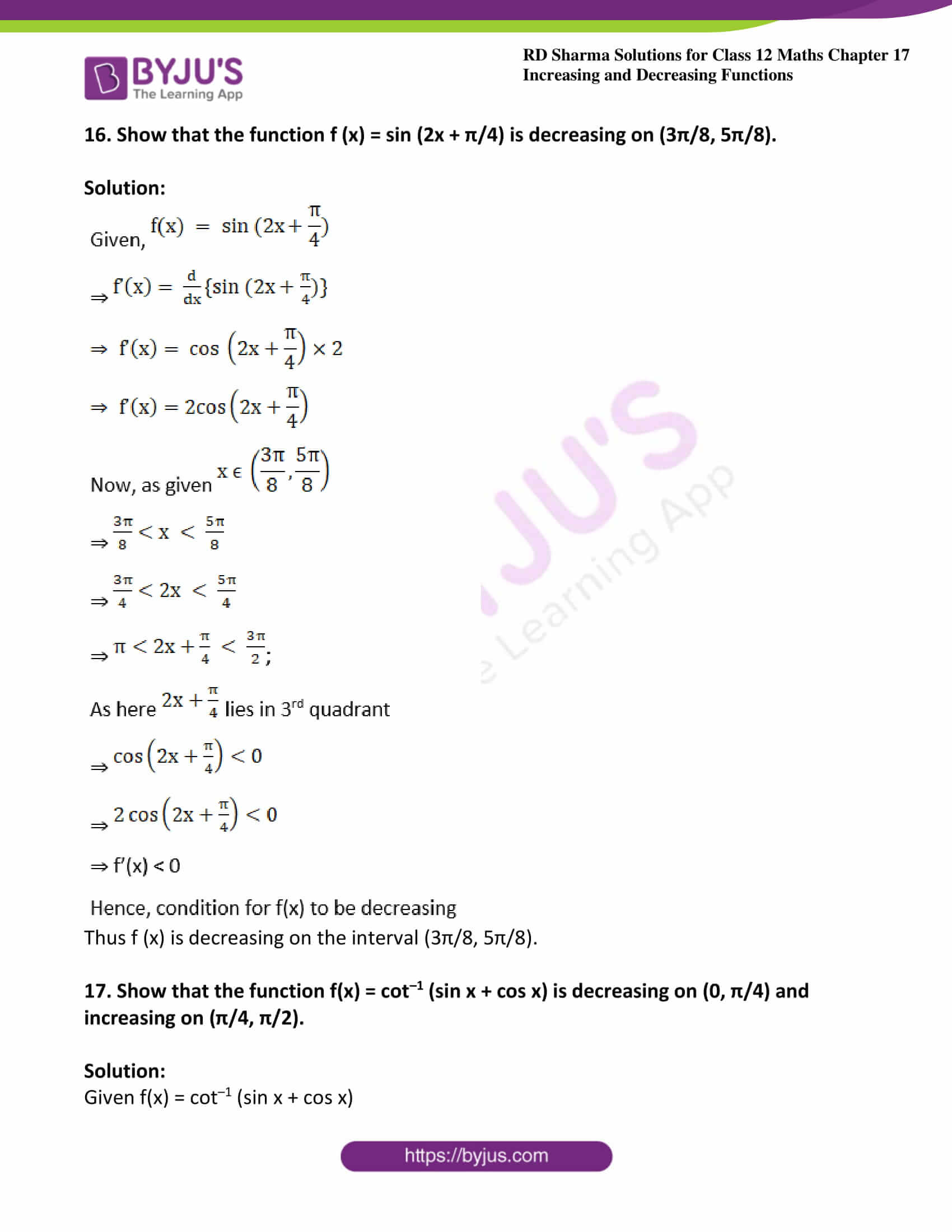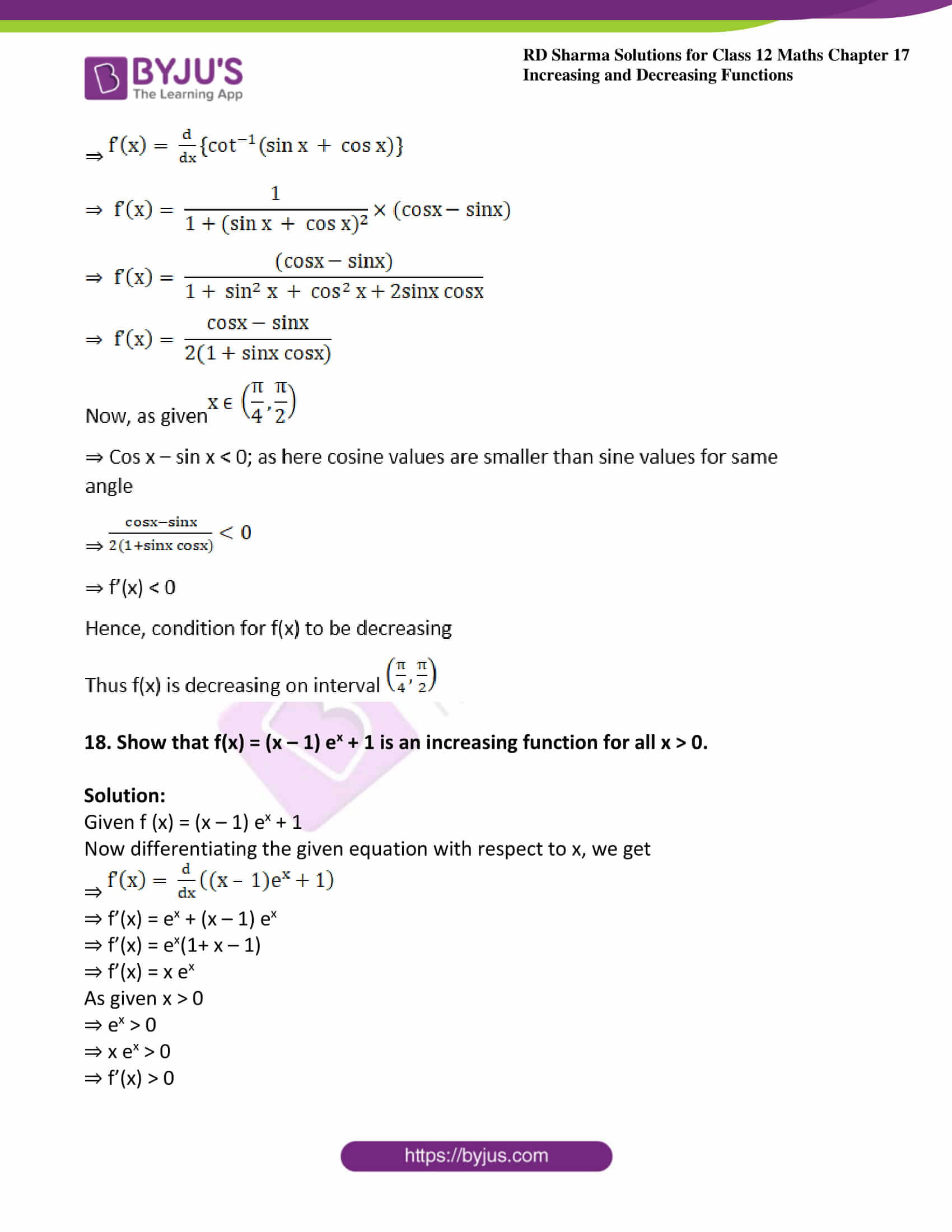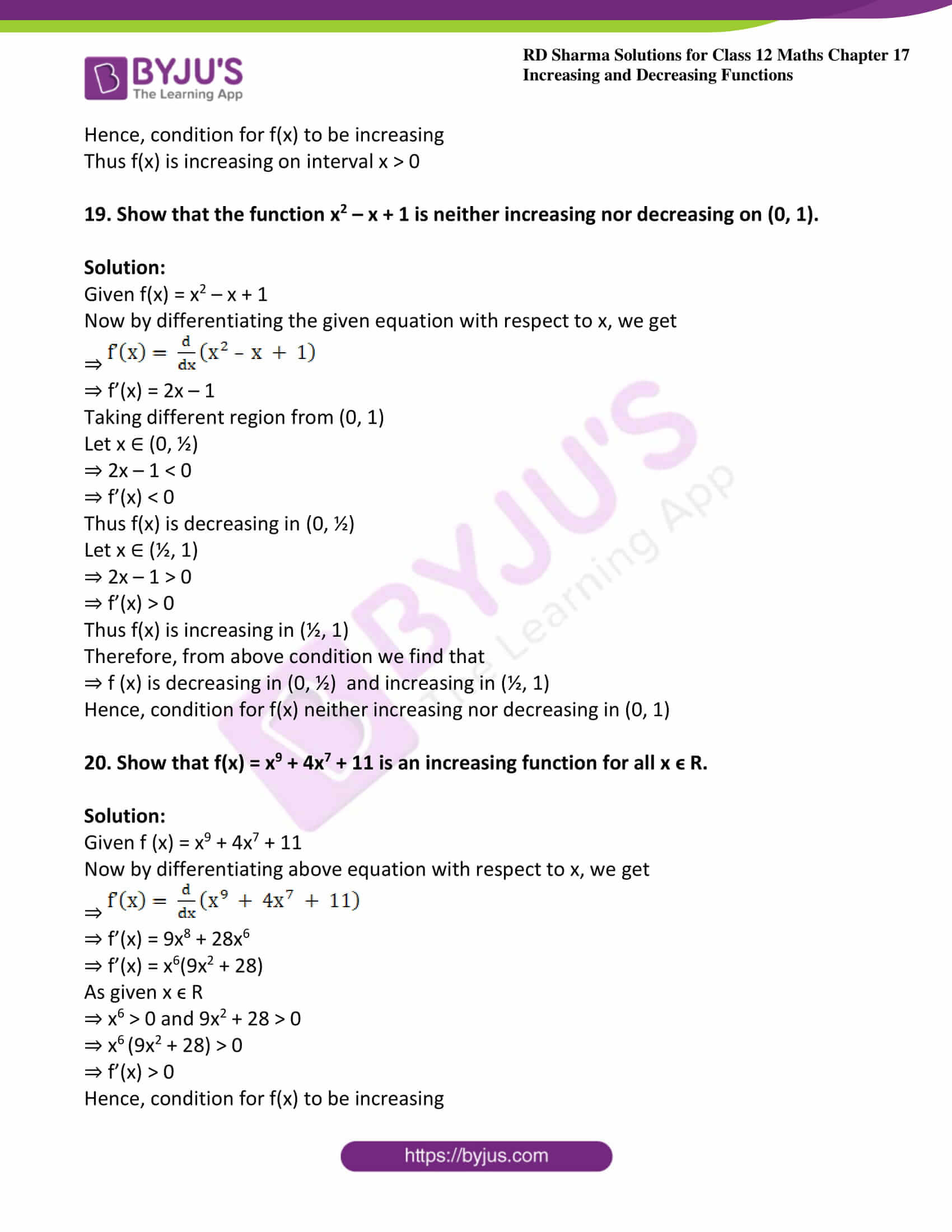### Access answers to Maths RD Sharma Solutions For Class 12 Chapter 17 – Increasing and Decreasing Functions

Exercise 17.1 Page No: 17.10

1. Prove that the function f(x) = loge x is increasing on (0, ∞).

Solution:

Let x1, x2 ∈ (0, ∞)

We have, x1 < x2

⇒ loge x1 < loge x2

⇒ f (x1) < f (x2)

So, f(x) is increasing in (0, ∞)

2. Prove that the function f(x) = loga x is increasing on (0, ∞) if a > 1 and decreasing on (0, ∞), if 0 < a < 1.

Solution: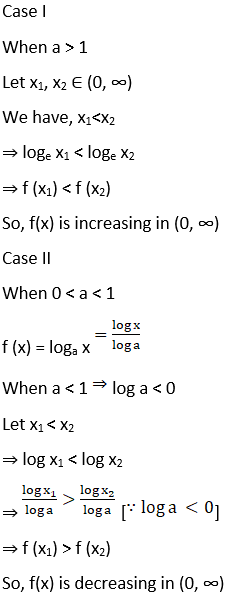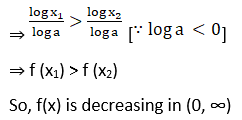3. Prove that f(x) = ax + b, where a, b are constants and a > 0 is an increasing function on R.

Solution:

Given,

f (x) = ax + b, a > 0

Let x1, x2 ∈ R and x1 > x2

⇒ ax1 > ax2 for some a > 0

⇒ ax1 + b> ax2 + b for some b

⇒ f (x1) > f(x2)

Hence, x1 > x2 ⇒ f(x1) > f(x2)

So, f(x) is increasing function of R

4. Prove that f(x) = ax + b, where a, b are constants and a < 0 is a decreasing function on R.

Solution:

Given,

f (x) = ax + b, a < 0

Let x1, x2 ∈ R and x1 > x2

⇒ ax1 < ax2 for some a > 0

⇒ ax1 + b < ax2 + b for some b

⇒ f (x1) < f(x2)

Hence, x1 > x2⇒ f(x1) < f(x2)

So, f(x) is decreasing function of R

Exercise 17.2 Page No: 17.33

1. Find the intervals in which the following functions are increasing or decreasing.

(i) f (x) = 10 – 6x – 2x2

Solution: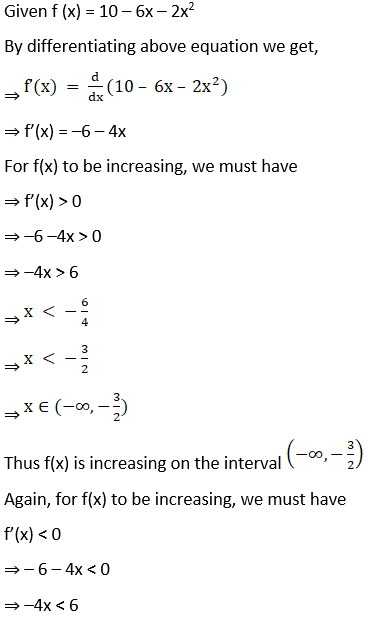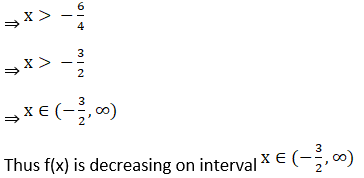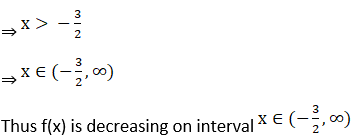(ii) f (x) = x2 + 2x – 5

Solution: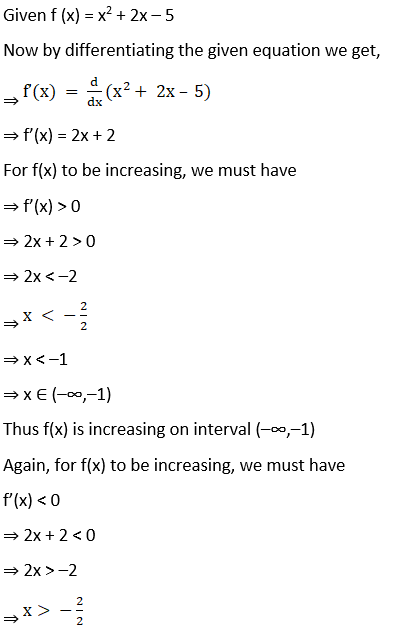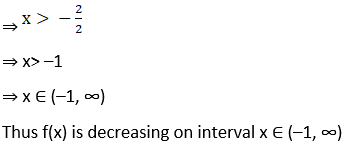(iii) f (x) = 6 – 9x – x2

Solution: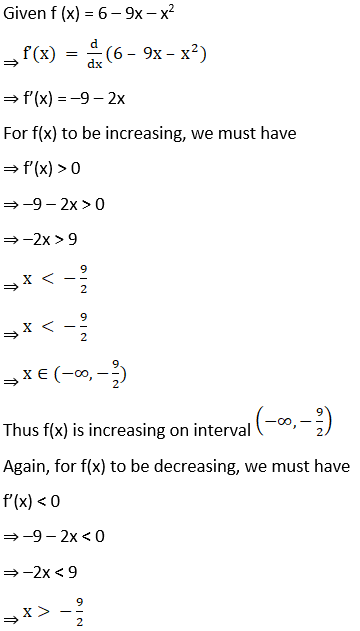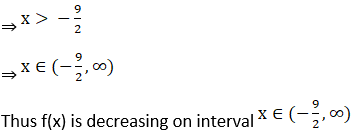(iv) f(x) = 2x3 – 12x2 + 18x + 15

Solution: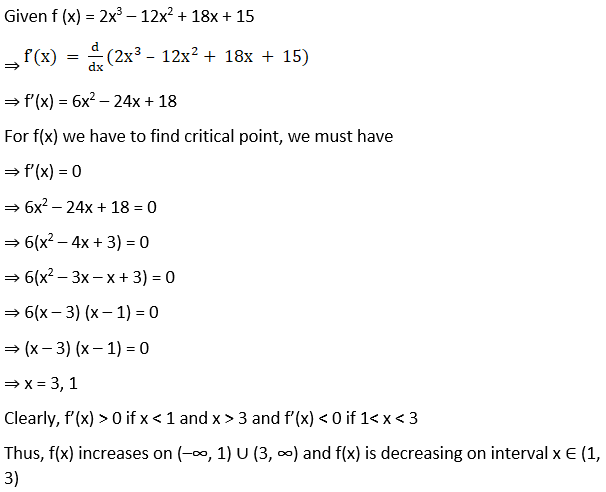(v) f (x) = 5 + 36x + 3x2 – 2x3

Solution:

Given f (x) = 5 + 36x + 3x2 – 2x3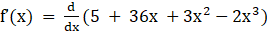⇒ f’(x) = 36 + 6x – 6x2

For f(x) now we have to find critical point, we must have

⇒ f’(x) = 0

⇒ 36 + 6x – 6x2 = 0

⇒ 6(–x2 + x + 6) = 0

⇒ 6(–x2 + 3x – 2x + 6) = 0

⇒ –x2 + 3x – 2x + 6 = 0

⇒ x2 – 3x + 2x – 6 = 0

⇒ (x – 3) (x + 2) = 0

⇒ x = 3, – 2

Clearly, f’(x) > 0 if –2< x < 3 and f’(x) < 0 if x < –2 and x > 3

Thus, f(x) increases on x ∈ (–2, 3) and f(x) is decreasing on interval (–∞, –2) ∪ (3, ∞)

(vi) f (x) = 8 + 36x + 3x2 – 2x3

Solution:

Given f (x) = 8 + 36x + 3x2 – 2x3

Now differentiating with respect to x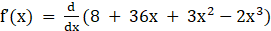⇒ f’(x) = 36 + 6x – 6x2

For f(x) we have to find critical point, we must have

⇒ f’(x) = 0

⇒ 36 + 6x – 6x2 = 0

⇒ 6(–x2 + x + 6) = 0

⇒ 6(–x2 + 3x – 2x + 6) = 0

⇒ –x2 + 3x – 2x + 6 = 0

⇒ x2 – 3x + 2x – 6 = 0

⇒ (x – 3) (x + 2) = 0

⇒ x = 3, – 2

Clearly, f’(x) > 0 if –2 < x < 3 and f’(x) < 0 if x < –2 and x > 3

Thus, f(x) increases on x ∈ (–2, 3) and f(x) is decreasing on interval (–∞, 2) ∪ (3, ∞)

(vii) f(x) = 5x3 – 15x2 – 120x + 3

Solution:

Given f(x) = 5x3 – 15x2 – 120x + 3

Now by differentiating above equation with respect x, we get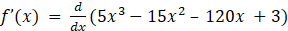⇒ f’(x) = 15x2 – 30x – 120

For f(x) we have to find critical point, we must have

⇒ f’(x) = 0

⇒ 15x2 – 30x – 120 = 0

⇒ 15(x2 – 2x – 8) = 0

⇒ 15(x2 – 4x + 2x – 8) = 0

⇒ x2 – 4x + 2x – 8 = 0

⇒ (x – 4) (x + 2) = 0

⇒ x = 4, – 2

Clearly, f’(x) > 0 if x < –2 and x > 4 and f’(x) < 0 if –2 < x < 4

Thus, f(x) increases on (–∞,–2) ∪ (4, ∞) and f(x) is decreasing on interval x ∈ (–2, 4)

(viii) f(x) = x3 – 6x2 – 36x + 2

Solution:

Given f (x) = x3 – 6x2 – 36x + 2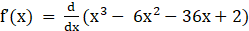⇒ f’(x) = 3x2 – 12x – 36

For f(x) we have to find critical point, we must have

⇒ f’(x) = 0

⇒ 3x2 – 12x – 36 = 0

⇒ 3(x2 – 4x – 12) = 0

⇒ 3(x2 – 6x + 2x – 12) = 0

⇒ x2 – 6x + 2x – 12 = 0

⇒ (x – 6) (x + 2) = 0

⇒ x = 6, – 2

Clearly, f’(x) > 0 if x < –2 and x > 6 and f’(x) < 0 if –2< x < 6

Thus, f(x) increases on (–∞,–2) ∪ (6, ∞) and f(x) is decreasing on interval x ∈ (–2, 6)

(ix) f(x) = 2x3 – 15x2 + 36x + 1

Solution:

Given f (x) = 2x3 – 15x2 + 36x + 1

Now by differentiating above equation with respect x, we get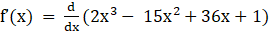⇒ f’(x) = 6x2 – 30x + 36

For f(x) we have to find critical point, we must have

⇒ f’(x) = 0

⇒ 6x2 – 30x + 36 = 0

⇒ 6 (x2 – 5x + 6) = 0

⇒ 6(x2 – 3x – 2x + 6) = 0

⇒ x2 – 3x – 2x + 6 = 0

⇒ (x – 3) (x – 2) = 0

⇒ x = 3, 2

Clearly, f’(x) > 0 if x < 2 and x > 3 and f’(x) < 0 if 2 < x < 3

Thus, f(x) increases on (–∞, 2) ∪ (3, ∞) and f(x) is decreasing on interval x ∈ (2, 3)

(x) f (x) = 2x3 + 9x2 + 12x + 20

Solution:

Given f (x) = 2x3 + 9x2 + 12x + 20

Differentiating above equation we get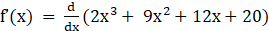⇒ f’(x) = 6x2 + 18x + 12

For f(x) we have to find critical point, we must have

⇒ f’(x) = 0

⇒ 6x2 + 18x + 12 = 0

⇒ 6(x2 + 3x + 2) = 0

⇒ 6(x2 + 2x + x + 2) = 0

⇒ x2 + 2x + x + 2 = 0

⇒ (x + 2) (x + 1) = 0

⇒ x = –1, –2

Clearly, f’(x) > 0 if –2 < x < –1 and f’(x) < 0 if x < –1 and x > –2

Thus, f(x) increases on x ∈ (–2,–1) and f(x) is decreasing on interval (–∞, –2) ∪ (–2, ∞)

2. Determine the values of x for which the function f(x) = x2 – 6x + 9 is increasing or decreasing. Also, find the coordinates of the point on the curve y = x2 – 6x + 9 where the normal is parallel to the line y = x + 5.

Solution:

Given f(x) = x2 – 6x + 9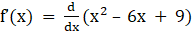⇒ f’(x) = 2x – 6

⇒ f’(x) = 2(x – 3)

For f(x) let us find critical point, we must have

⇒ f’(x) = 0

⇒ 2(x – 3) = 0

⇒ (x – 3) = 0

⇒ x = 3

Clearly, f’(x) > 0 if x > 3 and f’(x) < 0 if x < 3

Thus, f(x) increases on (3, ∞) and f(x) is decreasing on interval x ∈ (–∞, 3)

Now, let us find coordinates of point

Equation of curve is f(x) = x2 – 6x + 9

Slope of this curve is given by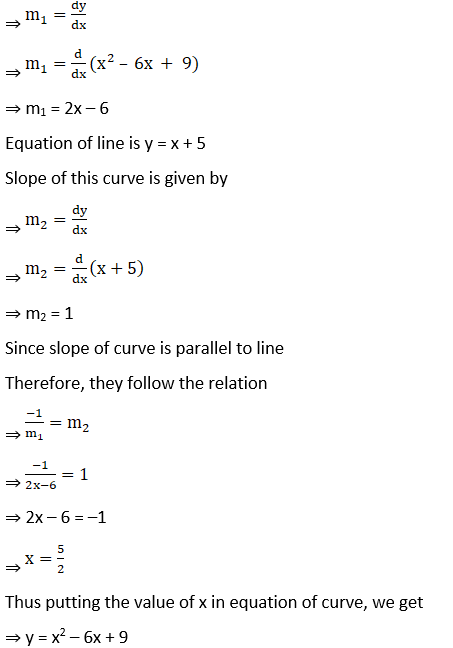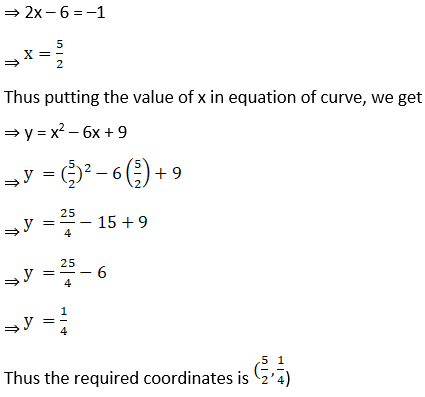3. Find the intervals in which f(x) = sin x – cos x, where 0 < x < 2π is increasing or decreasing.

Solution: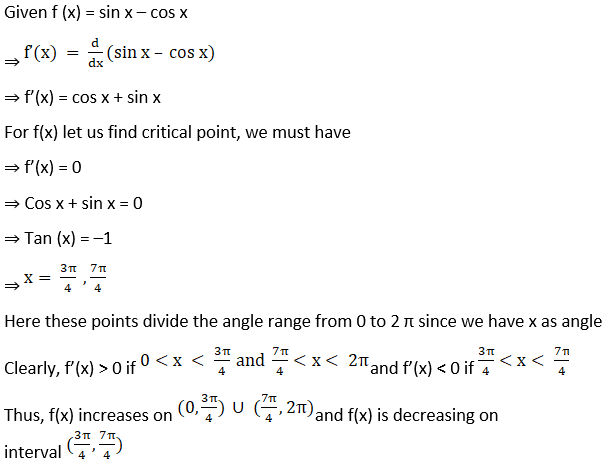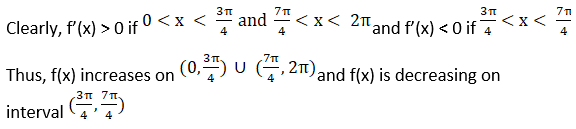4. Show that f(x) = e2x is increasing on R.

Solution:

Given f (x) = e2x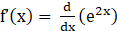⇒ f’(x) = 2e2x

For f(x) to be increasing, we must have

⇒ f’(x) > 0

⇒ 2e2x > 0

⇒ e2x > 0

Since, the value of e lies between 2 and 3

So, whatever be the power of e (that is x in domain R) will be greater than zero.

Thus f(x) is increasing on interval R

5. Show that f (x) = e1/x, x ≠ 0 is a decreasing function for all x ≠ 0.

Solution: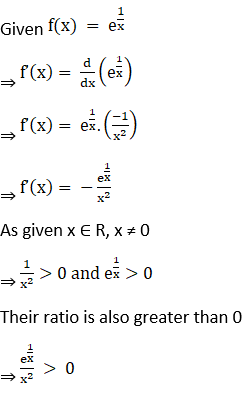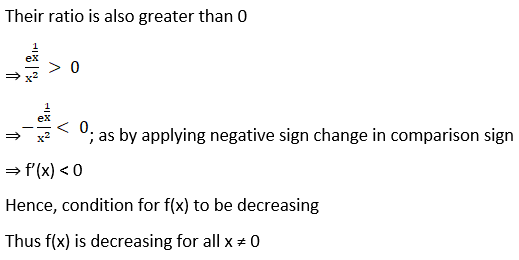6. Show that f(x) = loga x, 0 < a < 1 is a decreasing function for all x > 0.

Solution: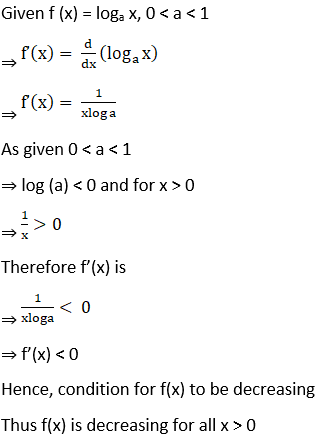7. Show that f(x) = sin x is increasing on (0, π/2) and decreasing on (π/2, π) and neither increasing nor decreasing in (0, π).

Solution: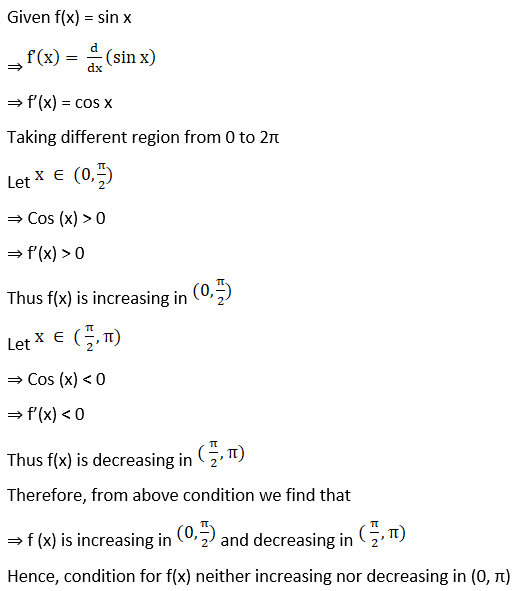8. Show that f(x) = log sin x is increasing on (0, π/2) and decreasing on (π/2, π).

Solution: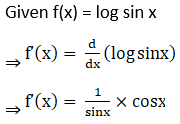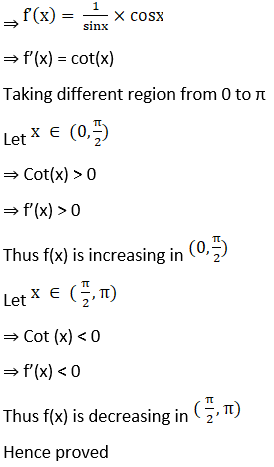9. Show that f(x) = x – sin x is increasing for all x ϵ R.

Solution:

Given f (x) = x – sin x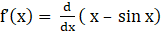⇒ f’(x) = 1 – cos x

Now, as given x ϵ R

⇒ –1 < cos x < 1

⇒ –1 > cos x > 0

⇒ f’(x) > 0

Hence, condition for f(x) to be increasing

Thus f(x) is increasing on interval x ∈ R

10. Show that f(x) = x3 – 15x2 + 75x – 50 is an increasing function for all x ϵ R.

Solution:

Given f(x) = x3 – 15x2 + 75x – 50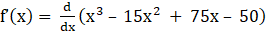⇒ f’(x) = 3x2 – 30x + 75

⇒ f’(x) = 3(x2 – 10x + 25)

⇒ f’(x) = 3(x – 5)2

Now, as given x ϵ R

⇒ (x – 5)2 > 0

⇒ 3(x – 5)2 > 0

⇒ f’(x) > 0

Hence, condition for f(x) to be increasing

Thus f(x) is increasing on interval x ∈ R

11. Show that f(x) = cos2 x is a decreasing function on (0, π/2).

Solution:

Given f (x) = cos2 x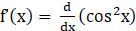⇒ f’(x) = 2 cos x (–sin x)

⇒ f’(x) = –2 sin (x) cos (x)

⇒ f’(x) = –sin2x

Now, as given x belongs to (0, π/2).

⇒ 2x ∈ (0,π)

⇒ Sin (2x)> 0

⇒ –Sin (2x) < 0

⇒ f’(x) < 0

Hence, condition for f(x) to be decreasing

Thus f(x) is decreasing on interval (0, π/2).

Hence proved

12. Show that f(x) = sin x is an increasing function on (–π/2, π/2).

Solution:

Given f (x) = sin x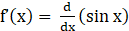⇒ f’(x) = cos x

Now, as given x ∈ (–π/2, π/2).

⇒ Cos x> 0

⇒ f’(x) > 0

Hence, condition for f(x) to be increasing

Thus f(x) is increasing on interval (–π/2, π/2).

13. Show that f(x) = cos x is a decreasing function on (0, π), increasing in (–π, 0) and neither increasing nor decreasing in (–π, π).

Solution:

Given f(x) = cos x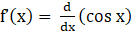⇒ f’(x) = –sin x

Taking different region from 0 to 2π

Let x ∈ (0, π).

⇒ Sin(x) > 0

⇒ –sin x < 0

⇒ f’(x) < 0

Thus f(x) is decreasing in (0, π)

Let x ∈ (–π, o).

⇒ Sin (x) < 0

⇒ –sin x > 0

⇒ f’(x) > 0

Thus f(x) is increasing in (–π, 0).

Therefore, from above condition we find that

⇒ f (x) is decreasing in (0, π) and increasing in (–π, 0).

Hence, condition for f(x) neither increasing nor decreasing in (–π, π)

14. Show that f(x) = tan x is an increasing function on (–π/2, π/2).

Solution:

Given f (x) = tan x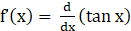⇒ f’(x) = sec2x

Now, as given

x ∈ (–π/2, π/2).

⇒ sec2x > 0

⇒ f’(x) > 0

Hence, Condition for f(x) to be increasing

Thus f(x) is increasing on interval (–π/2, π/2).

15. Show that f(x) = tan–1 (sin x + cos x) is a decreasing function on the interval (π/4, π /2).

Solution: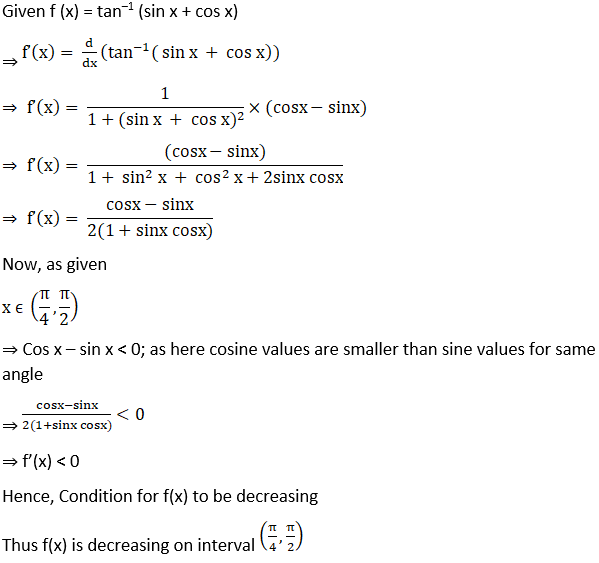16. Show that the function f (x) = sin (2x + π/4) is decreasing on (3π/8, 5π/8).

Solution: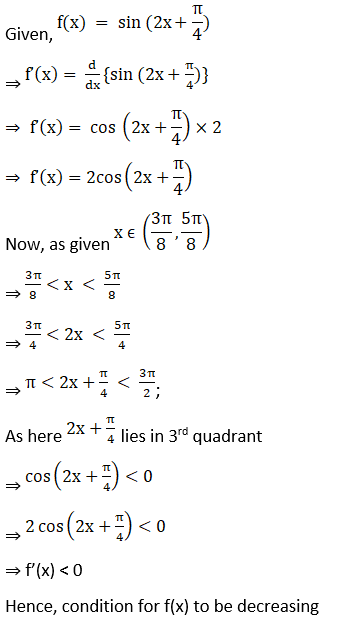Thus f (x) is decreasing on the interval (3π/8, 5π/8).

17. Show that the function f(x) = cot–1 (sin x + cos x) is decreasing on (0, π/4) and increasing on (π/4, π/2).

Solution:

Given f(x) = cot–1 (sin x + cos x)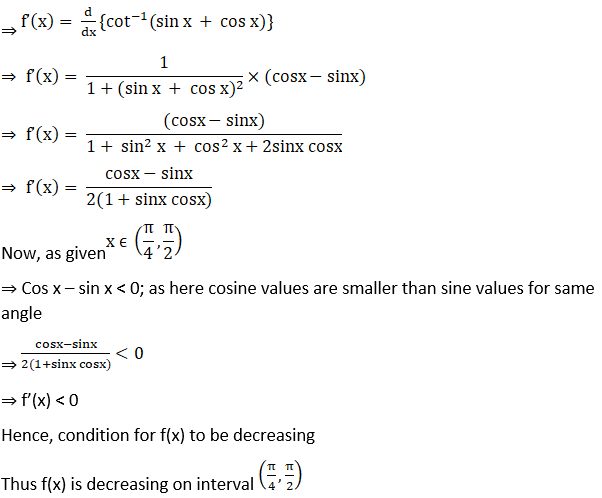18. Show that f(x) = (x – 1) ex + 1 is an increasing function for all x > 0.

Solution:

Given f (x) = (x – 1) ex + 1

Now differentiating the given equation with respect to x, we get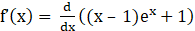⇒ f’(x) = ex + (x – 1) ex

⇒ f’(x) = ex(1+ x – 1)

⇒ f’(x) = x ex

As given x > 0

⇒ ex > 0

⇒ x ex > 0

⇒ f’(x) > 0

Hence, condition for f(x) to be increasing

Thus f(x) is increasing on interval x > 0

19. Show that the function x2 – x + 1 is neither increasing nor decreasing on (0, 1).

Solution:

Given f(x) = x2 – x + 1

Now by differentiating the given equation with respect to x, we get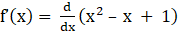⇒ f’(x) = 2x – 1

Taking different region from (0, 1)

Let x ∈ (0, ½)

⇒ 2x – 1 < 0

⇒ f’(x) < 0

Thus f(x) is decreasing in (0, ½)

Let x ∈ (½, 1)

⇒ 2x – 1 > 0

⇒ f’(x) > 0

Thus f(x) is increasing in (½, 1)

Therefore, from above condition we find that

⇒ f (x) is decreasing in (0, ½)  and increasing in (½, 1)

Hence, condition for f(x) neither increasing nor decreasing in (0, 1)

20. Show that f(x) = x9 + 4x7 + 11 is an increasing function for all x ϵ R.

Solution:

Given f (x) = x9 + 4x7 + 11

Now by differentiating above equation with respect to x, we get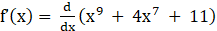⇒ f’(x) = 9x8 + 28x6

⇒ f’(x) = x6(9x2 + 28)

As given x ϵ R

⇒ x6 > 0 and 9x2 + 28 > 0

⇒ x6 (9x2 + 28) > 0

⇒ f’(x) > 0

Hence, condition for f(x) to be increasing

Thus f(x) is increasing on interval x ∈ R

### Also, access RD Sharma Solutions for Class 12 Maths Chapter 17 Increasing and Decreasing Functions

Exercise 17.1 Solutions

Exercise 17.2 Solutions

## Frequently Asked Questions on RD Sharma Solutions for Class 12 Maths Chapter 17

### How many exercises are present in RD Sharma Solutions for Class 12 Maths Chapter 17?

There are 2 exercises are present in RD Sharma Solutions for Class 12 Maths Chapter 17 Viz,
Exercise 17.1
Exercise 17.2

### RD Sharma Solutions for Class 12 Maths Chapter 17 enough for board exam preparation?

Yes, RD Sharma Solutions for Class 12 Maths Chapter 17 provides solutions for all questions given in RD Sharma Textbook Maths for Class 12. Most of the questions in the exams are asked from these exercises. By learning these concepts, you can score high in your finals.

### What are the main topics covered in RD Sharma Solutions for Class 12 Maths Chapter 17?

The solution of rational algebraic inequations
Strictly increasing functions
Strictly decreasing functions
Monotonic functions
Monotonically increasing function
Monotonically decreasing functions
Necessary and sufficient conditions for monotonicity
Finding the intervals in which a function is increasing or decreasing
Proving the monotonicity of a function on a given interval
Finding the interval in which a function is increasing or decreasing
By practicing all these exercises you will be able to attend all the questions related to Increasing and Decreasing Functions in examinations.